Simple n-bit analog to digital converter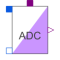# Information

This information is part of the Modelica Standard Library maintained by the Modelica Association.

Simple analog to digital converter with a variable resolution of n bits. It converts the input voltage `ppin.v-npin.v` to an n-vector of type Logic (9-valued logic according to IEEE 1164 STD_ULOGIC). The input resistance between positive and negative pin is determined by `Rin`. Further effects (like input capacities) have to be modeled outside the converter, since this should be a general model.

The input signal range (VRefLo,VRefHi) is divided into 2^n-1 equally spaced stages of length Vlsb:=(VRefHi-VRefLo)/(2^n-1). The output signal is the binary code of ` k ` as long as the input voltage takes values in the k-th stage, namely in the range from `Vlsb*(k-0.5)` to `m*(k+0.5)`. This is called mid-tread operation. Additionally the output can only change its value if the trigger signal `trig` of type Logic changes to '1' (forced or weak).

The output vector is a 'little-endian'. i.e., that the first bit y is the least significant one (LSB).

This is an abstract model of an ADC. Therefore, it can not cover the dynamic behaviour of the converter. Hence the output will change instantaneously when the trigger signal rises.

# Parameters (4)

N Value: Type: Integer Description: Resolution in bits - output signal width Value: Type: Voltage (V) Description: High reference voltage Value: Type: Voltage (V) Description: Low reference voltage Value: Type: Resistance (Ω) Description: Input resistance

# Connectors (4)

p n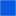Type: PositivePin Description: Positive electrical pin (input)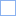Type: NegativePin Description: Negative electrical pin (input)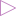Type: DigitalOutput[N] Description: Digital output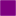Type: DigitalInput Description: Trigger input

# Used in Examples (1)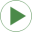AD_DA_conversion Modelica.Electrical.Analog.Examples Conversion circuit